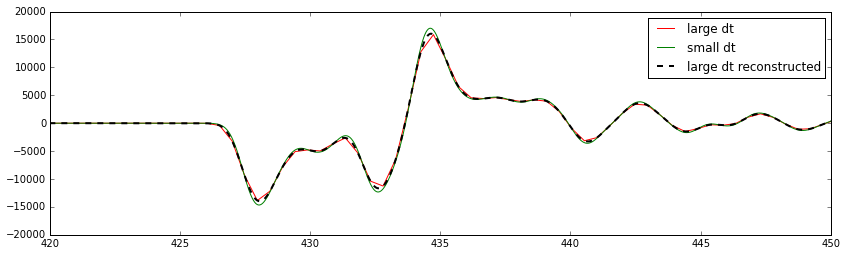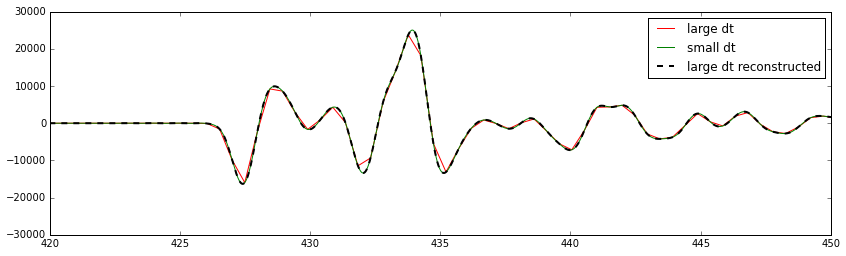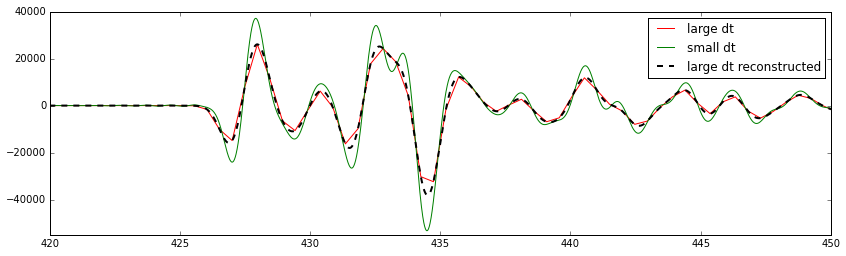## Consequences of the order of resampling and differentiation/integration¶

The current version of the syngine service by default returns seismograms with the sampling rate of the database. That sampling rate is naturally as low as possible to save disc space. If people request velocity seismograms everything is good. When people request either displacement or acceleration we have to either numerically integrate or differentiate. These operations are not accurate and perform at their worst for frequencies close to Nyquist.

Thus significant errors are introduced if people just requests seismograms (in displacement/acceleration) without manually specifying a smaller dt. For large dt values Instaseis internally will resample before it performs the differentiation/integration and all is good.

Only very few people will be aware of that and to avoid people requesting bad seismograms I propose to set the default dt to a tenth of the database sampling interval. The data is then effectively oversampled by a factor of 10 which puts more load on IRIS's connection but the error is no longer an issue.

In all the following examples the green line and the dashed black ones should be identical.

In :
%matplotlib inline
import obspy
import requests
import matplotlib.pyplot as plt


### Displacement¶

The error is fairly small as it only performs an integration.

In :
large_dt = obspy.read("http://service.iris.edu/irisws/syngine/1/query?"
"network=IU&station=ANMO&components=Z&eventid=GCMT:M110302J&format=miniseed&"
"units=displacement")
"network=IU&station=ANMO&components=Z&eventid=GCMT:M110302J&format=miniseed&"
"dt=0.01&kernelwidth=16&units=displacement")

large_dt_reconstructed = large_dt.copy().interpolate(100.0, method="lanczos", window="blackman", a=16)

plt.figure(figsize=(14, 4))
plt.plot(large_dt.times(), large_dt.data, color="red", label="large dt")
plt.plot(small_dt.times(), small_dt.data, color="green", label="small dt")
plt.plot(large_dt_reconstructed.times(), large_dt_reconstructed.data,
"k--", label="large dt reconstructed", lw=2)
plt.legend()
plt.xlim(420, 450)
plt.ylim(-20000, 20000)
plt.show()### Velocity¶

No error as database effectively stores velocity.

### No error for velocity as no differentiation/integration has to be performed¶

In :
large_dt = obspy.read("http://service.iris.edu/irisws/syngine/1/query?"
"network=IU&station=ANMO&components=Z&eventid=GCMT:M110302J&format=miniseed&"
"units=velocity")
"network=IU&station=ANMO&components=Z&eventid=GCMT:M110302J&format=miniseed&"
"dt=0.01&kernelwidth=16&units=velocity")

large_dt_reconstructed = large_dt.copy().interpolate(100.0, method="lanczos", window="blackman", a=16)

plt.figure(figsize=(14, 4))
plt.plot(large_dt.times(), large_dt.data, color="red", label="large dt")
plt.plot(small_dt.times(), small_dt.data, color="green", label="small dt")
plt.plot(large_dt_reconstructed.times(), large_dt_reconstructed.data,
"k--", label="large dt reconstructed", lw=2)
plt.legend()
plt.xlim(420, 450)
plt.ylim(-30000, 30000)
plt.show()### Acceleration¶

Very large error. The used central difference operator acts as a very strong lowpass filter that filters frequency depending on the current sampling rate of the signal. See e.g. here: http://www.holoborodko.com/pavel/numerical-methods/numerical-derivative/central-differences/

In :
large_dt = obspy.read("http://service.iris.edu/irisws/syngine/1/query?"
"network=IU&station=ANMO&components=Z&eventid=GCMT:M110302J&format=miniseed&"
"units=acceleration")
"network=IU&station=ANMO&components=Z&eventid=GCMT:M110302J&format=miniseed&"
"dt=0.01&kernelwidth=16&units=acceleration")

large_dt_reconstructed = large_dt.copy().interpolate(100.0, method="lanczos", window="blackman", a=16)

plt.figure(figsize=(14, 4))
plt.plot(large_dt.times(), large_dt.data, color="red", label="large dt")
plt.plot(small_dt.times(), small_dt.data, color="green", label="small dt")
plt.plot(large_dt_reconstructed.times(), large_dt_reconstructed.data,
"k--", label="large dt reconstructed", lw=2)
plt.legend()
plt.xlim(420, 450)
plt.ylim(-55000, 40000)
plt.show()